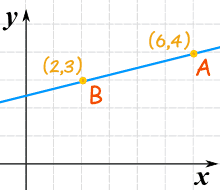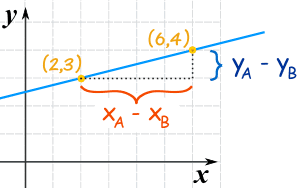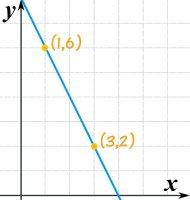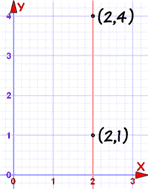# 从两点找线的方程12单位向右 5单位向上

## 从两点找坡度（斜率）### 这条线的坡度（斜率）是什么？

• 点 "A" 是 (6, 4) （当 y 是 4，x 是 6）
• 点 "B" 是 (2, 3) （当 y 是 3，x 是 2）。。。。。。。公式是： 坡度 m  =  y 的改变x 的改变  =  yA − yBxA − xB

• 计算Y值的差，
• 计算X值的差
• 相除

m  =   y 的改变 x 的改变   =   4−3 6−2   =   1 4 = 0.25

m  =   y 的改变 x 的改变   =   3−4 2−6   =   −1 −4 = 0.25

## 已知两点求方程### 这条线的方程是什么？

y − y1 = m(x − x1)

y − 3 = m(x − 2)

y − 3 = (1/4)(x − 2)

y − 3 = x/4 − 2/4

y = x/4 − ½ + 3

y = x/4 + 5/2

### 检测！

y = x/4 + 5/2 = 6/4 + 2.5 = 1.5 + 2.5 = 4

## 另一个例子### 这条线的方程是什么？

"点斜式" 开始：

y − y1 = m(x − x1)

• x1 = 1
• y1 = 6
• m = (2−6)/(3−1) = −4/2 = −2

y − 6 = −2(x − 1)

y − 6 = −2x + 2

y = −2x + 8

## 重要的例外垂直线的斜率是未定义的（因为我们不能除以零）： m = yA − yBxA − xB = 4 − 12 − 2 = 30 = 未定义 但我们仍然可以写这个方程：用 x=，而不用 y=，像这样： x = 2